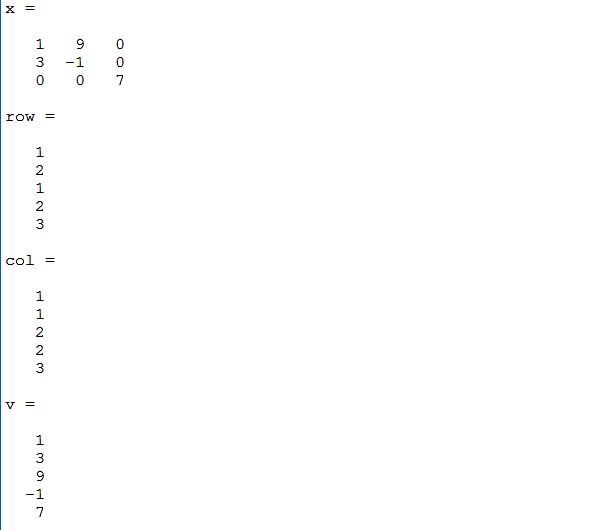Open In App

# How to Find Index of Element in Array in MATLAB?

In MATLAB, the arrays are used to represent the information and data. You can use indexing to access the elements of the array.  In MATLAB the array indexing starts from 1. To find the index of the element in the array, you can use the find() function. Using the find() function you can find the indices and the element from the array. The find() function returns a vector containing the data.

Syntax:

• find(X) : Return a vector containing the indices of elements
• find(X,n): Return first n indices of the elements in X
• find(X,n, Direction): find n indices in X according to the Direction  where Direction – ‘first‘ or ‘last
• [row,col] = find(): It returns the row and column subscript of element in array
• [row,col,V] = find(): returns vector V containing non-zero elements

Now let’s see how to find an index of any element in an array using the find() function with the help of examples.

### find(x)

find(X) returns a vector containing the linear indices of each nonzero element in array X.

Example 1:

## Matlab

 `% MATLAB code for  find an index of any ``% element in an array using the find()``array = [1 2 3 4 5 6]`` ` `% find() will get the index of element``% store it in the index``index = find(array==3)`

Output: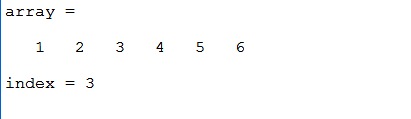Note: If the array contains duplicates then find(X) function will return all the indices of that integer.

Example 2:

## Matlab

 `% MATLAB code for if  the array contains``% duplicate elements ``array = [1 2 3 4 5 6 2 4 2]`` ` `% find() will get the index of element``% store it in the index``index = find(array==2)`

Output: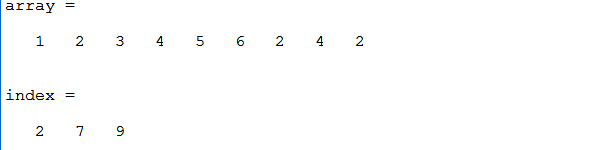When the array contains duplicate values the find() function will print all the indices of that corresponding element. So if you don’t want all the indices of that element you can use the find(X,n) function.

### find(X,n)

Return first n indices of the elements in X.

Example:

## Matlab

 `% MATLAB code for return first ``% n indices of the elements in X``array = [1 2 3 4 5 6 2 4 2]`` ` `% find() will get the index of element``% gets the first index of 2``% store it in the index``index = find(array==2,1)`

Output: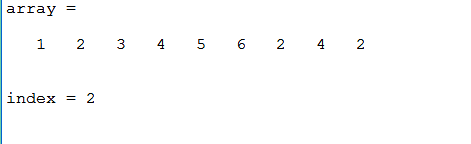### find(X,n,Direction)

You can also find the index of the elements from both directions in the array. Both directions mean from starting and from last by using find(X,n,Direction). This function find n indices in X according to the Direction. The Direction parameter accepts either ‘first’ or ‘last’. If the direction is first it will return first n indices of that corresponding element or if the direction is last it will return the indices by traversing from the end of the array. By default, the Direction parameter is ‘first’.

Example 1:

## Matlab

 `% MATLAB code for find the index of ``% the elements from both directions ``% in the array``array = [1 2 3 4 5 6 2 4 2]`` ` `% find() will get the index of element``% store it in the index``index = find(array==2,2,``'first'``)`

Output: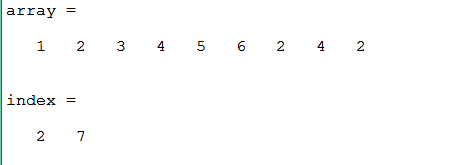Example 2:

## Matlab

 `% array of integers``array = [1 2 3 4 5 6 2 4 2]`` ` `% find() will get the index of element``% store it in the index``index = find(array==2,2,``'last'``)`

Output: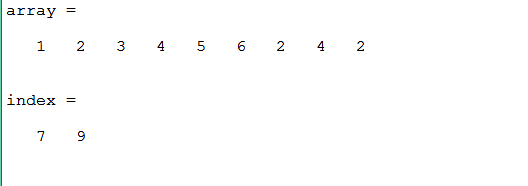### [row,col] = find(x)

For finding the index of an element in a 3-Dimensional array you can use the syntax [row,col] = find(x) this will give you the row and the column in which the element is present.

Example:

## Matlab

 `% MATLAB code for Finding an index ``% of an element in a 3-D array``array = [1 2 3; 4 5 6; 7 8 9]`` ` `% find() will get the index of element``% prints the row and column of the element``[row,col] = find(array==5)`

Output: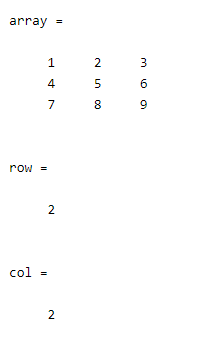### [row,col,v] = find(X)

If you want to find the indices of all the non-zero elements present in the 3-dimensional array you can use [row,col,v] = find(X) where X is our array. This will find all indices of all non-zero elements present in the array and store them into the vector v

Example:

## Matlab

 `% MATLAB code for find the indices of ``% all the non-zero elements present in the 3-D array ``x = [1 9 0; 3 -1 0; 0 0 7]`` ` `% find() will get the indices of the elements``% and the vector will store all the non-zero elements``[row,col,v] = find(x)`

Output: